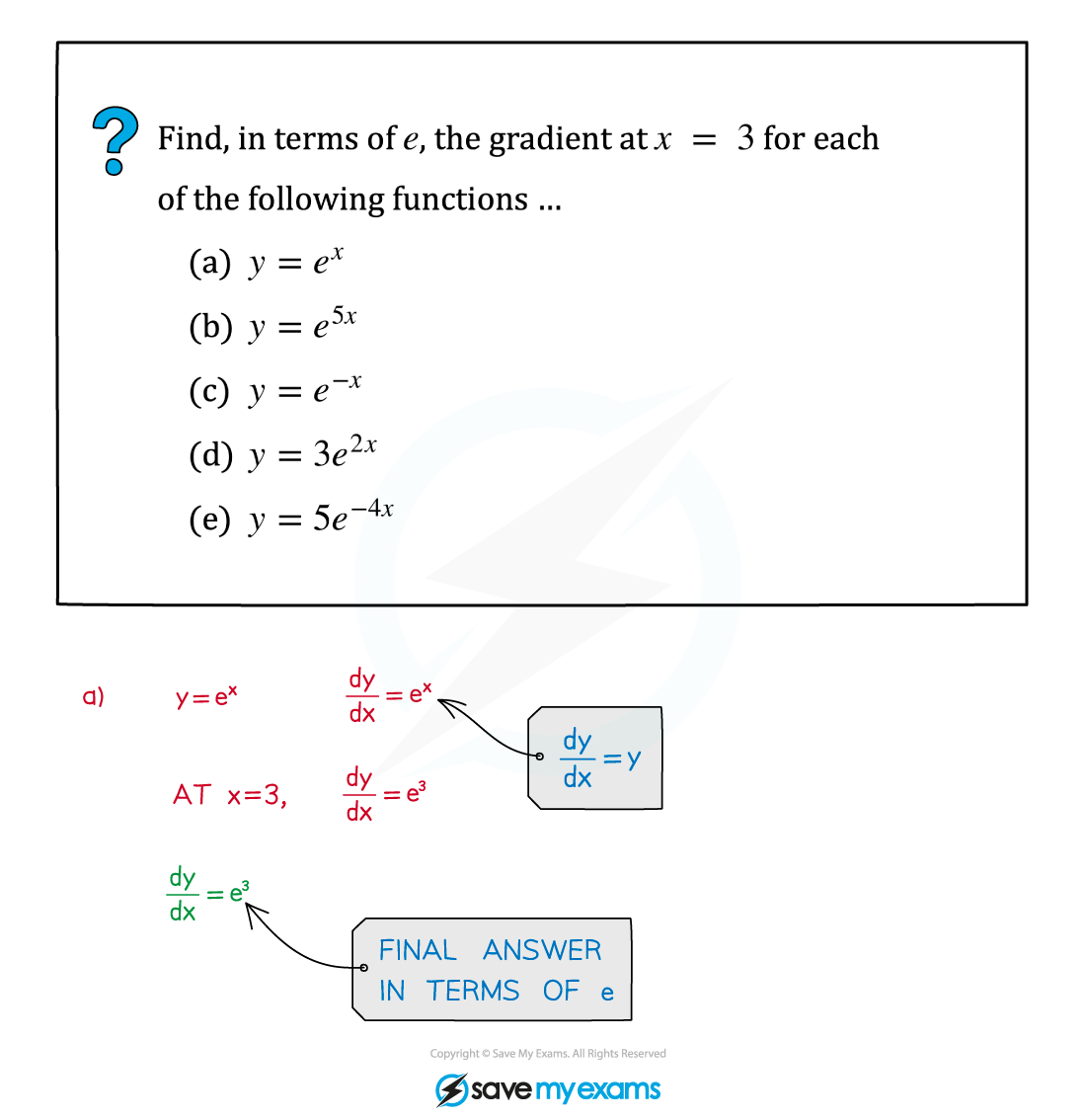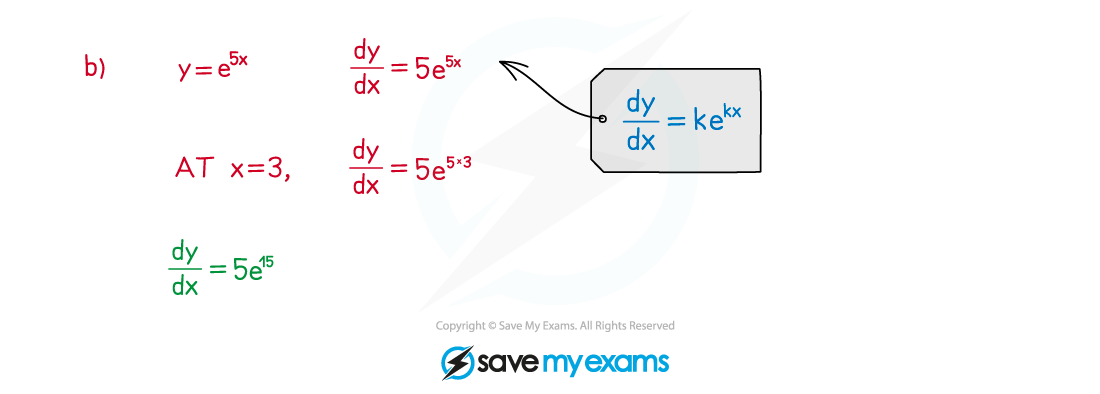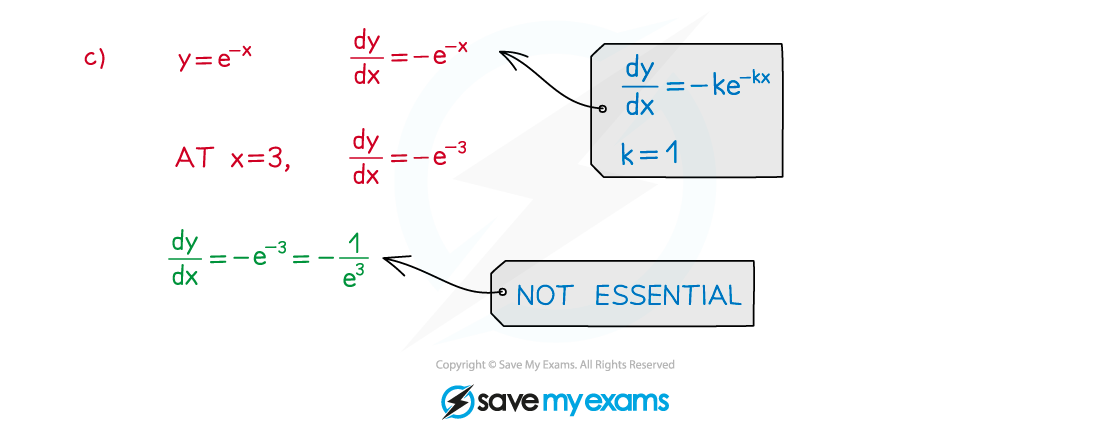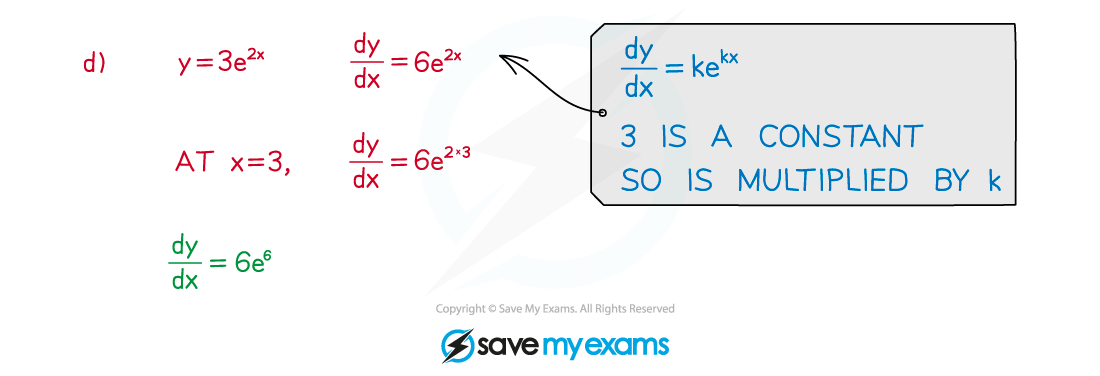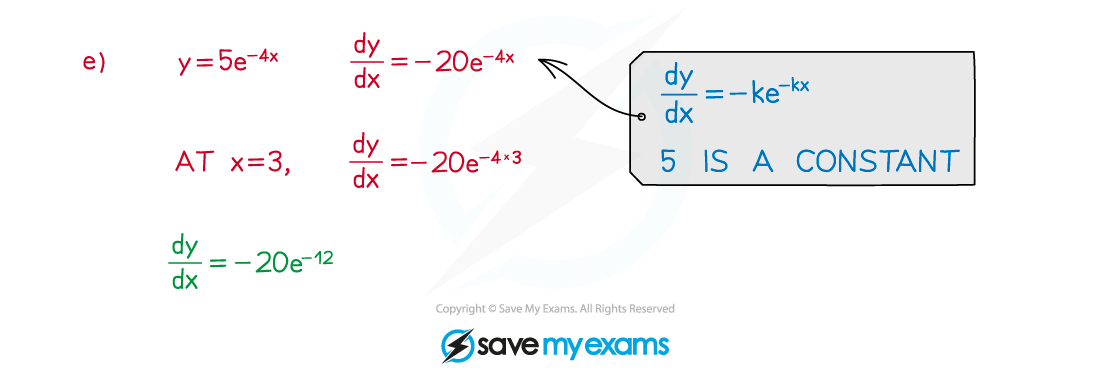# AQA A Level Maths: Pure复习笔记6.1.4 Derivatives of Exponential Functions

### Derivatives of Exponential Functions

#### What is the derivative of e?

• y = ex has the particular property
•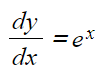ie for every real number x, the gradient of y = ex is also equal to e(see Derivatives of Exponential Functions)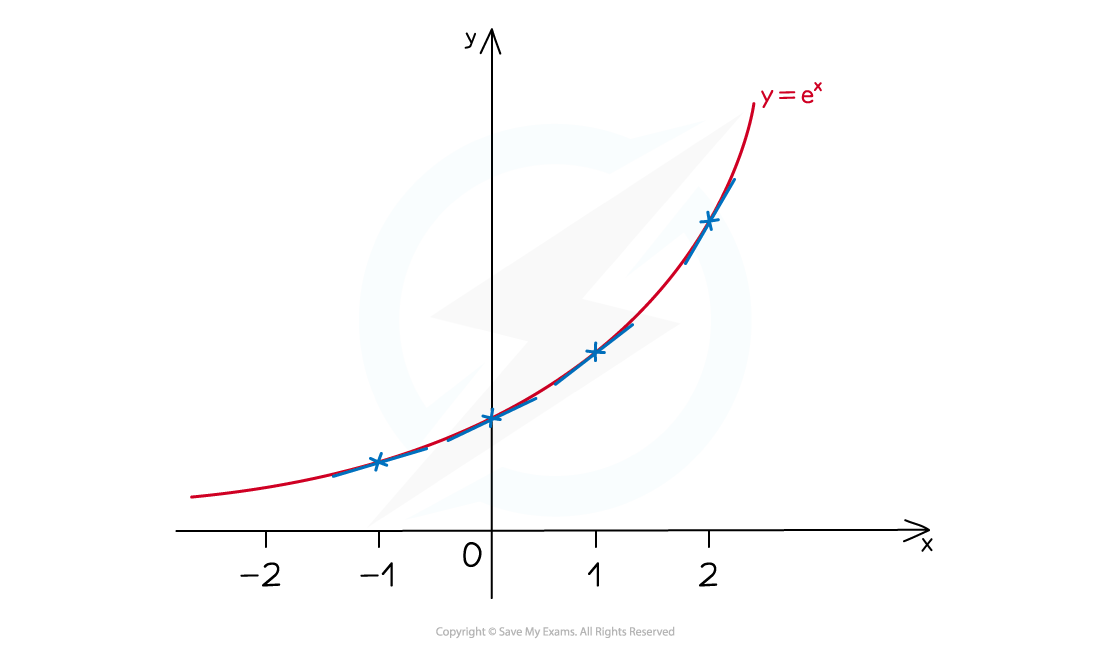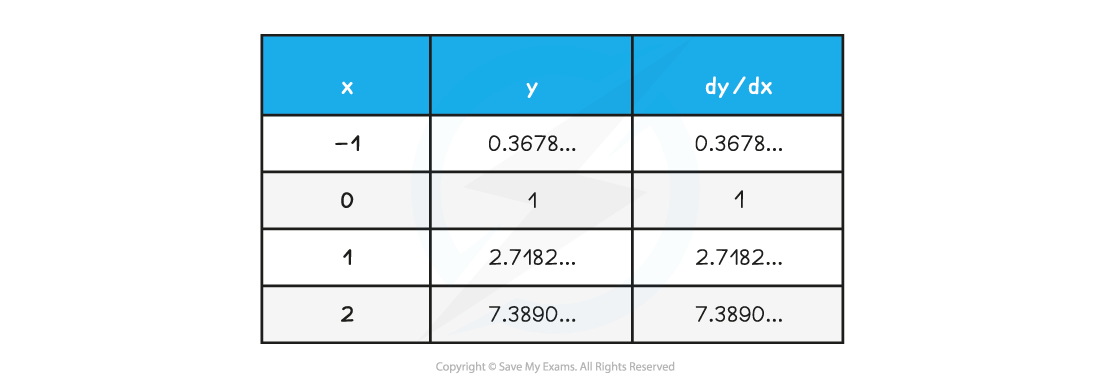• e ≈ 2.718 (see "e")
• Recall that the derivative is the gradient function for a curve (see First Principles Differentiation)

#### Graphs and derivatives related to e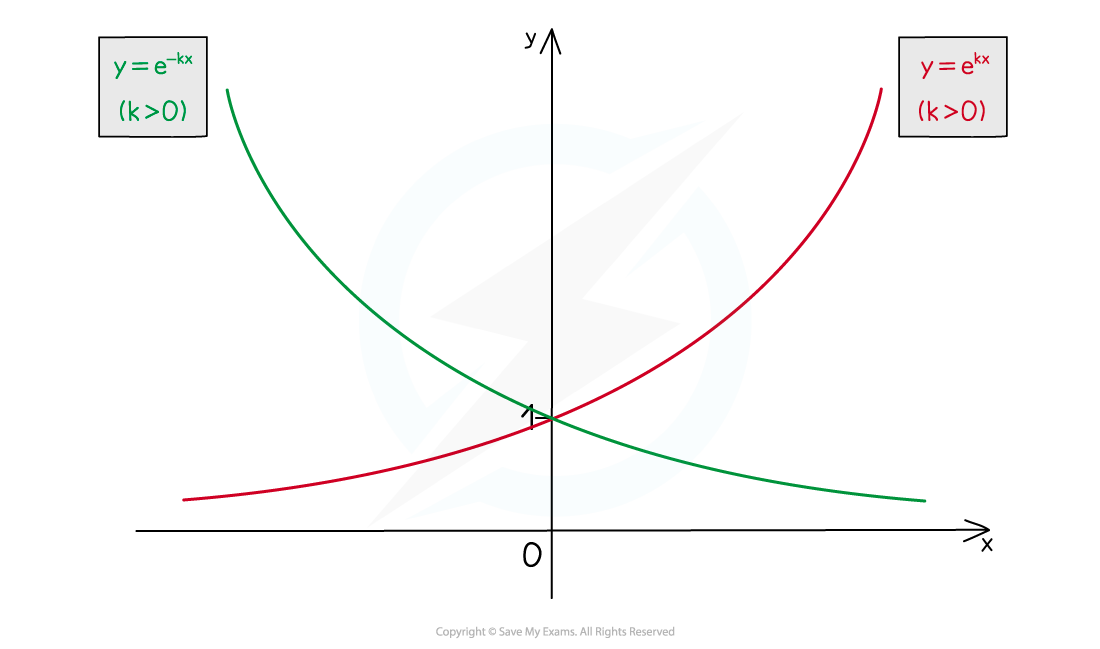•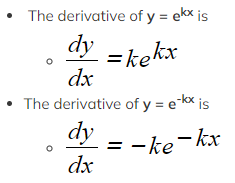Exam Tip
• Remember that (like πe is a number.
• Exam questions can ask for answers to be given as exact values in terms of e (see the Worked Example below).

#### Worked Example